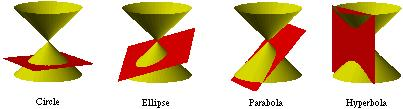index: click on a letter A B C D E F G H I J K L M N O P Q R S T U V W X Y Z A to Z index index: subject areas numbers & symbols sets, logic, proofs geometry algebra trigonometry advanced algebra & pre-calculus calculus advanced topics probability & statistics real world applications multimedia entrieswww.mathwords.com about mathwords website feedback

 Conic Sections The family of curves including circles, ellipses, parabolas, and hyperbolas. All of these geometric figures may be obtained by the intersection a double cone with a plane, hence the name conic section. All conic sections have equations of the form Ax2 + Bxy + Cy2 + Dx + Ey + F = 0.See also